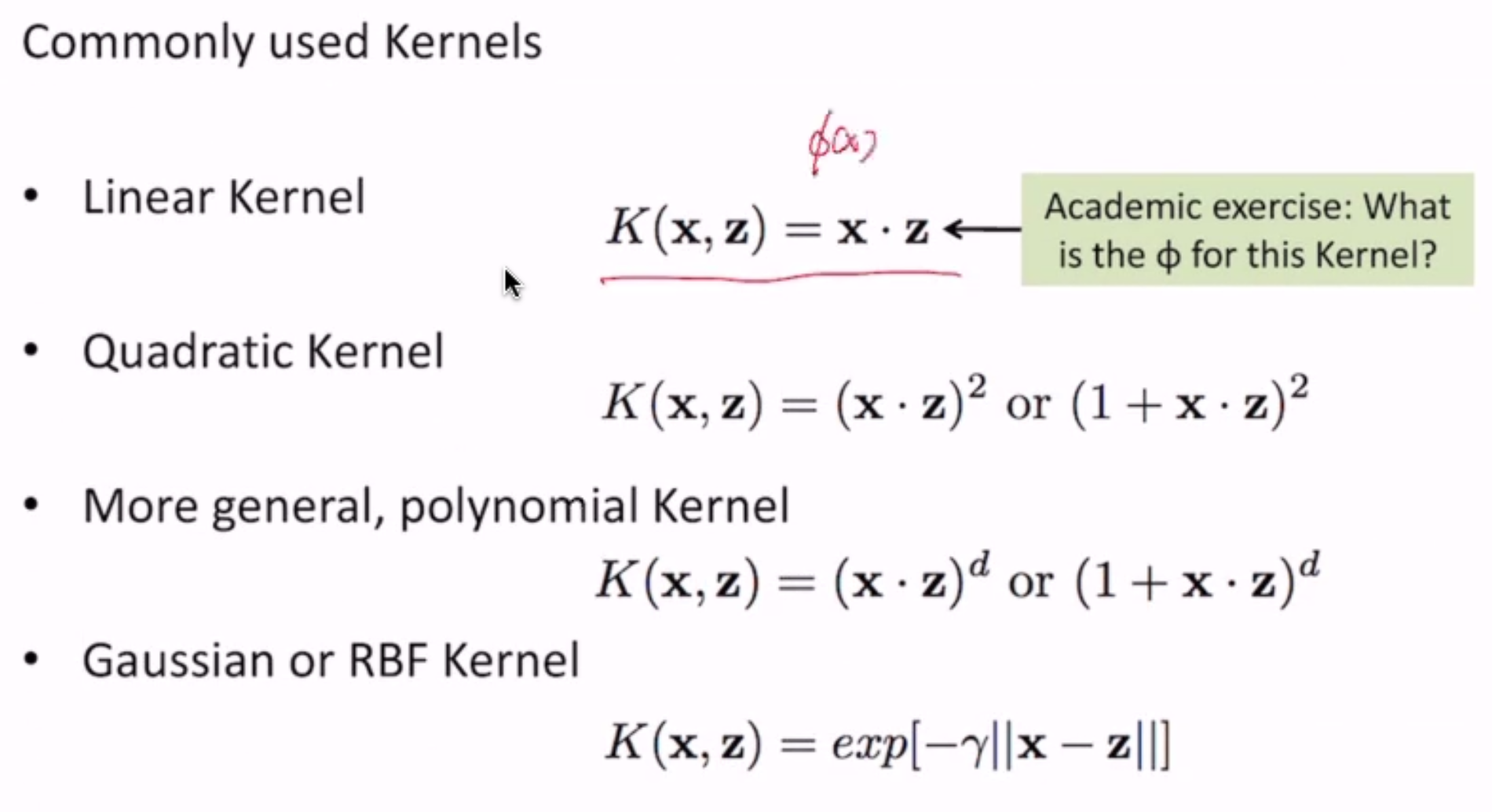# Kernel Methods¶

• step 1: use a special type of mapping functions
• still map feature space to a higher dimensional space
• but computation of $$\phi(x) \cdot \phi(z)$$ is easy (remember the multiplication of features in SVM optimization)
• step 2: rewrite the model s.t.
• the mapping $$\phi(x)$$ never needs to be explicitly computed - i.e. never compute terms like $$w \cdot \phi(x) + b$$
• only work with $$\phi(x) \cdot \phi(z)$$ - we call this the kernel function

Ex.

$\mathbf{x} = <x_1, x_2> \to \phi(x) = <x_1^2, \sqrt{2}x_1 x_2, x_2^2>$

We can compute $$\phi(x) \cdot \phi(z)$$ easily:

$\begin{split}\phi(x) \cdot \phi(z) & = <x_1^2, \sqrt{2}x_1 x_2, x_2^2> \\ & = <x_1^2, \sqrt{2}x_1 x_2, x_2^2> \cdot <z_1^2, \sqrt{2}z_1 z_2, z_2^2> \\ & = ... \\ & = (x \cdot z)^2 \\ & = K(x, z)\end{split}$

We call this a kernel function

$... = (1+x \cdot z)^2 = K(x, z)$

## Kernel in SVM¶

Rewriting the primal form of the SVM to use the mapped space doesn’t seem easy.

But we can rewrite the dual form easily!

$\begin{split}\max & \sum_{n=1}^N \alpha_n - \frac{1}{2} \sum_{m,n=1}^N \alpha_m \alpha_n y_m y_n (\phi(x_m)^T \phi(x_n)) \\ \text{subject to } & \sum_{n=1}^N \alpha_n y_n = 0, \alpha_n \geq 0; n = 1..N\end{split}$

Kernelized SVM

Now SVM is computing a linear boundary in the higher-dimensional space without actually transforming vectors

$\begin{split}\max & \sum_{n=1}^N \alpha_n - \frac{1}{2} \sum_{m,n=1}^N \alpha_m \alpha_n y_m y_n (K(x_m, x_n)) \\ \text{subject to } & \sum_{n=1}^N \alpha_n y_n = 0, \alpha_n \geq 0; n = 1..N\end{split}$

Predictions:

$\begin{split}\hat{y} & = sign(\sum \alpha_n y_n x_n \cdot x' + b) \text{ (in the old space)} \\ & \to sign(\sum \alpha_n y_n \phi(x_n) \cdot \phi(x') + b) \\ & = sign(\sum \alpha_n y_n K(x_n, x') + b)\end{split}$

## Formal Definition¶

Let’s use the example kernel $$K(x, z) = (x \cdot z)^2$$ for $$\phi(x) = <x_1^2, \sqrt{2}x_1x_2, x_2^2>$$

• a kernel function is implicitly associated with some $$\phi$$
• $$\phi$$ maps input $$x\in X$$ to a higher dimensional space $$F$$:
• $$\phi: X \to F$$
• kernel takes 2 inputs from $$X$$ and outputs their similarity in F
• $$K: X \times X \to R$$
• once you have a kernel, you don’t need to know $$\phi$$

Mercer’s Condition

• a function can be a kernel function K if a suitable $$\phi$$ exists
• $$\exists \phi$$ s.t. $$K(x, z) = \phi(x) \cdot \phi(z)$$
• mathematically: K should be positive semi-definite; i.e. for all square integrable f s.t. $$\int f(x)^2 dx < \infty$$
• $$\int \int f(x)K(x, z)f(z)dxdz > 0$$
• sufficient and necessary to identify a kernel function

Constructing Kernels

We already know some proven basic kernel functions - given Mercer’s condition, we can construct new kernels!

• $$k(x, z) = k_1(x, z) + k_2(x, z)$$ (direct sum)
• $$k(x, z) = \alpha k_1(x, z)$$ ($$\forall \alpha > 0$$ - scalar product)

Note

Example: given that k1 and k2 are kernels, prove $$k(x, z) = k_1(x, z) + k_2(x, z)$$ is a kernel

$\begin{split}\iint f(x)K(x, z)f(z)dxdz & = \iint f(x)[K_1(x, z) + K_2(x, z)]f(z)dxdz \\ & = \iint f(x)K_1(x, z)f(z)dxdz + \iint f(x)K_2(x, z)f(z)dxdz \\ & > 0 + 0\end{split}$($$\phi$$ for the linear kernel = $$\phi(x) = x$$)

## Perceptron¶

we can also apply kernels to perceptron!

Naively, we can just replace $$\mathbf{x}$$ with $$\phi(x)$$ in the algorithm - but that requires knowledge of $$\phi$$Prediction

since $$w = \sum_m \alpha_m \phi(x_m)$$, prediction on $$x_n$$ is easy:

$\hat{y_n} = sign(\sum_m \alpha_m \phi(x_m) \cdot \phi(x_n) + b)$